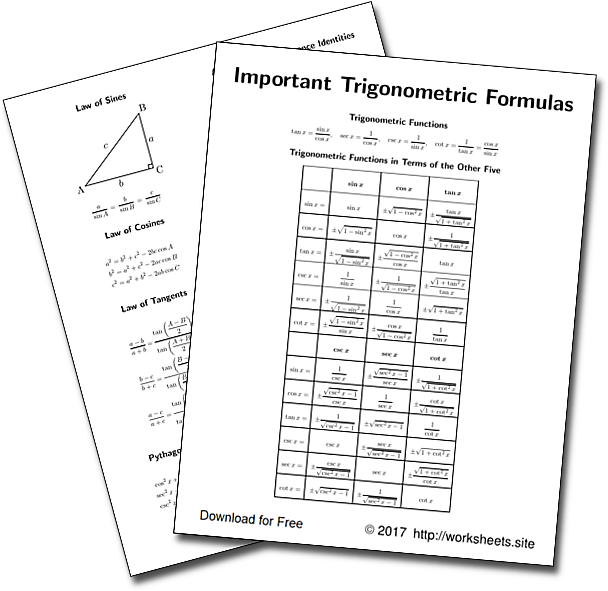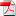# Trig Cheat Sheet

Free Trigonometry CheatSheet. Compilation of all trigonometric identities such as those found in Trigonometry books. Trig Formula Sheet to print on a single sheet side and side. Trig Conversion sheet. Trig Functions cheat sheet.The PDF file has four pages to print on both sides of the same sheet with the basic relationships of trigonometry. Use the worksheets.site password to open the PDFfile. Once the sheet is printed on both sides, it should be folded in half, so you will have a small booklet or book. The PDF file has two pages to print on both sides of a single sheet.

This leaflet will help you solve trig problems and make trigonometry homework easier.

1. Trigonometric functions in terms of the Other Five
2. Common Angles
3. Symmetry, Shifts and Periodicity
4. Calculus of Trigonometric Function
5. Fundamental Properties and Formulas
6. Law of Sines
7. Law of Cosines
8. Law of Tangents
9. Pythagorean Identities
10. Sum and Difference of Angles
11. Angle Sum and Difference Identities
12. Product to Sum
13. Sum to Product
14. Double Angle Trigonometric Identities
15. Triple Angle Trigonometric Identities
16. Medium Angle Trigonometric Identities
17. Power Reduction Formulas
18. Difference of Squares to Product
19. Composition of Functions
20. Sum and Difference of Inverse Trigonometric Functions

In mathematics, the trigonometric functions are functions of an angle, important when studying triangles and modeling periodic phenomena. They are commonly defined as ratios of two sides of a right triangle containing the angle, and can equivalently be defined as the lengths of various line segments from a unit circle.

The trigonometric functions are of great importance in Physics, Astronomy, Cartography, Boating, Telecommunications, the representation of periodic phenomena, and many other applications.

A trigonometric identity is an equality between expressions that contain trigonometric functions and is valid for all the values of the angle in which the functions are defined.